# 1431 is a Triangular Number and a Hexagonal Number

If you only look at a list of triangular numbers or a list of hexagonal numbers, you might miss the relationship that figurate numbers have with each other.

1378 is the 52nd triangular number, and you can use it to find the 53rd triangular number (1431), the 53rd square number, the 53rd pentagonal number, and so forth.

351 is the 26th triangular number, and you can use it to find the 27th triangular number, the 27th square number, the 27th pentagonal number, the 27th hexagonal number (1431), and so forth.

See the relationship in the graphic below:Should you get excited that 1431 is BOTH a triangular number and a hexagonal number? Not really. It turns out that every hexagonal number is also a triangular number. (But not every triangular number is a hexagonal number.)

Here are a few more facts about the number 1431:

• 1431 is a composite number.
• Prime factorization: 1431 = 3 × 3 × 3 × 53, which can be written 1431 = 3³ × 53.
• 1431 has at least one exponent greater than 1 in its prime factorization so √1431 can be simplified. Taking the factor pair from the factor pair table below with the largest square number factor, we get √1431 = (√9)(√159) = 3√159.
• The exponents in the prime factorization are 3 and 1. Adding one to each exponent and multiplying we get (3 + 1)(1 + 1) = 4 × 2 = 8. Therefore 1431 has exactly 8 factors.
• The factors of 1431 are outlined with their factor pair partners in the graphic below.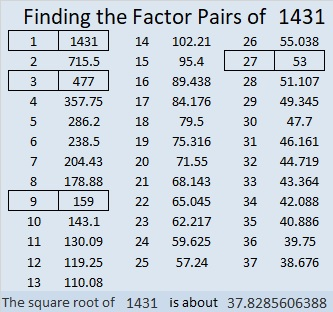1431 is the hypotenuse of a Pythagorean triple:
756-1215-1431 which is 27 times (28-45-53)

# The Shape of 1326

1326 is the 51st triangular number because 26 × 51 = 1326 and 2(26) is 1 more than 51.

Since 51 is an odd number, 1326 is also a hexagonal number. It is the 26th hexagonal number because 26 is half of 52 which is 1 more than 51.

Triangular numbers aren’t hard to visualize, but hexagonal numbers might be. Here are 1326 tiny dots showing that 1326 is a hexagonal number:Here are some more facts about the number 1326:

• 1326 is a composite number.
• Prime factorization: 1326 = 2 × 3 × 13 × 17
• The exponents in the prime factorization are 1, 1, 1, and 1. Adding one to each and multiplying we get (1 + 1)(1 + 1)(1 + 1)(1 + 1) = 2 × 2 × 2 × 2 = 16. Therefore 1326 has exactly 16 factors.
• Factors of 1326: 1, 2, 3, 6, 13, 17, 26, 34, 39, 51, 78, 102, 221, 442, 663, 1326
• Factor pairs: 1326 = 1 × 1326, 2 × 663, 3 × 442, 6 × 221, 13 × 102, 17 × 78, 26 × 51, or 34 × 39
• 1326 has no square factors that allow its square root to be simplified. √1326 ≈ 36.41428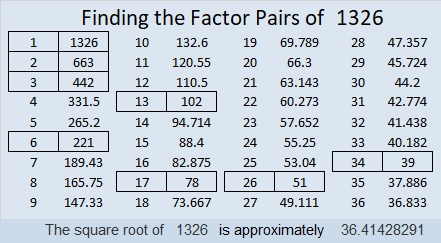Here are some factor trees for 1326 that won’t topple over.1326 is also the long side of four different Pythagorean triple triangles:
840-1026-1326 which is 6 times (140-171-221)
126-1320-1326 which is 6 times (21-220-221)
510-1224-1326 which is (5-12-13) times 102
624-1170-1326 which is (8-15-17) times 78

# 1225 is a Triangular Number, a Perfect Square, and . . .

1 and 36 are both triangular numbers and square numbers. The next number that can make the same claim is 1225. Why is this so?

1225 is a triangular number that just happens to be the sum of two consecutive triangular numbers. Two consecutive triangular numbers can always be made into a perfect square. Here’s a gif that tries to illustrate these facts about 1225:GIFs like this at MakeaGif

1225 is the 49th triangular number because 49(50)/2 =1225

It is the 35th square number because 35² = 1225

That it is both a triangular number AND a square number is pretty remarkable. But guess what? 1225 is ALSO a hexagonal number.

1225 is the 25th hexagonal number because (25)(2·25 – 1)= 1225
In fact, 1225 is the smallest number greater than 1 that is a triangle, a square, AND a hexagon! (Yeah, 1 is also all three and a whole more, but does 1 dot REALLY look like a triangle, a square, a hexagon and everything else all at the same time?)
All of these facts are great reasons to get very excited about the number 1225. Here are some more facts about this number:
• 1225 is a composite number.
• Prime factorization: 1225 = 5 × 5 × 7 × 7, which can be written 1225 = 5²× 7²
• The exponents in the prime factorization are 2 and 2. Adding one to each and multiplying we get (2 + 1)(2 + 1) = 3 × 3 = 9. Therefore 1225 has exactly 9 factors.
• Factors of 1225: 1, 5, 7, 25, 35, 49, 175, 245, 1225
• Factor pairs: 1225 = 1 × 1225, 5 × 245, 7 × 175, 25 × 49, or 35 × 35
• 1225 is a perfect square. √1225 = 35
Not only is 1225 a triangular number that is the sum of two other triangular numbers, but
1225 is also a square that is the sum of two other squares!
28² + 21² = 35² = 1225

1225 is the hypotenuse of two Pythagorean triples:
735-980-1225 which is (3-4-5) times 245
343-1176-1225 calculated from 28² – 21², 2(28)(21), 28² + 21²,
but it is also (7-24-25) times 49

1225 looks like a square in some other bases:
It’s 441 in BASE 17,
169 in BASE 32,
144 in BASE 33,
121 in BASE 34, and
100 in BASE 35

OEIS.org reports that 1225 is the smallest number that can be written as the sum of four cubes three different ways. It looks like these are the three ways:
10³ + 6 ³ + 2³ + 1³ = 1225
9³ + 6³ + 6³ + 4³ = 1225
8³ + 7³ + 7³ + 3³ = 1225

1225 is, indeed, the smallest number that has all the special properties listed above!

# 1035 is the 23rd Hexagonal Number

1035 is the 23rd hexagonal number because of the way that it can be factored:
2(23²) – 23 = 1035,
(2(23) – 1)23 = 1035
or simply 45(23) = 1035.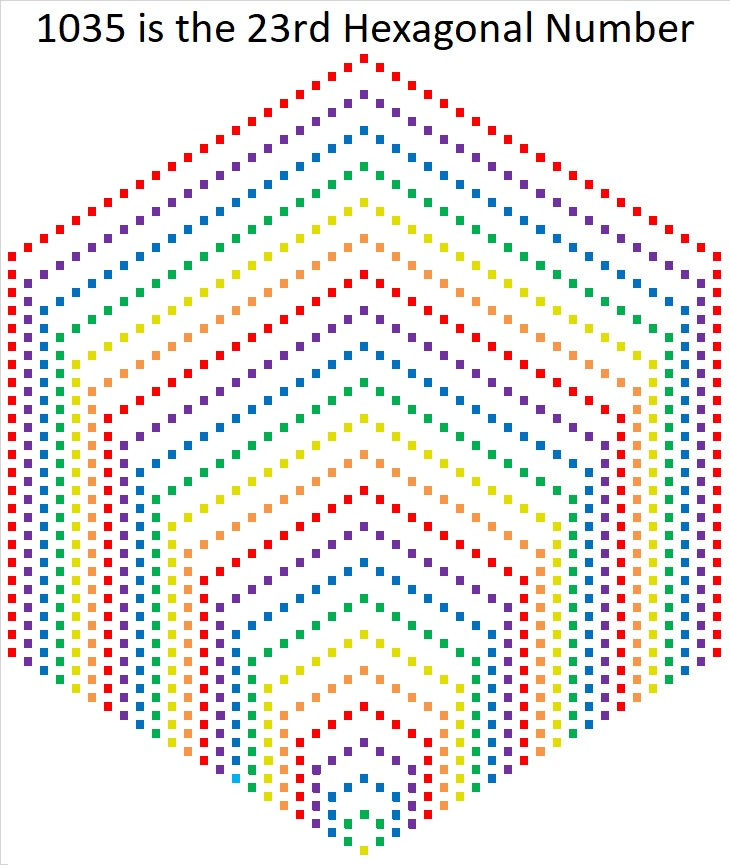Starting at the bottom of the hexagonal we see 1 yellow square, 5 green squares, 9 blue squares, 13 purple squares, 17 red squares, and 21 orange squares.

1, 5, 9, 13, 17, 21, . . . is an arithmetic progression or arithmetic sequence. The common difference between the numbers is 4.

The nth hexagonal number is the sum of the first n numbers in that arithmetic progression.
The first few hexagonal numbers form an arithmetic series: 1, 6, 15, 28, 45, 66 and so forth.
1035 is the sum of the first 23 numbers in the progression so it is the 23rd term in the series and the 23rd hexagonal number.

All hexagonal numbers are also triangular numbers. 1035 is the 45th triangular number because 45(46)/2 = 1035.Starting in the lower left-hand corner of that triangle we see 1 yellow square, 2 green squares, 3 blue squares, 4 purple squares, 5 red squares, and 6 orange squares.

1, 2, 3, 4, 5, 6, . . .  is the simplest arithmetic progression there is. The common difference is 1.

The nth triangular number is the sum of the first n numbers in that arithmetic progression.

The first few triangular numbers form an arithmetic series: 1, 3,  6, 10, 15, 21, 28, 36, 45, 55, 66 and so forth.  (The blue triangular numbers are also hexagonal numbers.)
1035 is the sum of the first 45 numbers in the progression so it is the 45th term in the series and the 45th triangular number.

1035 is also the hypotenuse of one Pythagorean triple:
621-828-1035 which is (3-4-5) times 207

It is also a leg in several Pythagorean triples including
1035-1380-1725 which is (3-4-5) times 345

• 1035 is a composite number.
• Prime factorization: 1035 = 3 × 3 × 5 × 23, which can be written 1035 = 3² × 5 × 23
• The exponents in the prime factorization are 2, 1, and 1. Adding one to each and multiplying we get (2 + 1)(1 + 1)(1 + 1) = 3 × 2 × 2 = 23. Therefore 1035 has exactly 12 factors.
• Factors of 1035: 1, 3, 5, 9, 15, 23, 45, 69, 115, 207, 345, 1035
• Factor pairs: 1035 = 1 × 1035, 3 × 345, 5 × 207, 9 × 115, 15 × 69, or 23 × 45,
• Taking the factor pair with the largest square number factor, we get √1035 = (√9)(√115) = 3√115 ≈ 32.1714# 780 is the 39th Triangular Number

• 780 is a composite number.
• Prime factorization: 780 = 2 x 2 x 3 x 5 x 13, which can be written 780 = (2^2) x 3 x 5 x 13
• The exponents in the prime factorization are 2, 1, 1, and 1. Adding one to each and multiplying we get (2 + 1)(1 + 1)(1 + 1)(1 + 1) = 2 x 3 x 2 x 2 = 24. Therefore 780 has exactly 24 factors.
• Factors of 780: 1, 2, 3, 4, 5, 6, 10, 12, 13, 15, 20, 26, 30, 39, 52, 60, 65, 78, 130, 156, 195, 260, 390, 780
• Factor pairs: 780 = 1 x 780, 2 x 390, 3 x 260, 4 x 195, 5 x 156, 6 x 130, 10 x 78, 12 x 65, 13 x 60, 15 x 52, 20 x 39, or 26 x 30
• Taking the factor pair with the largest square number factor, we get √780 = (√4)(√195) = 2√195 ≈ 27.92848.There are MANY ways to make factor trees for 780. Here are just three of them:OEIS.org informs us that (7 + 5)(8 + 5)(0 + 5) = 780.

780 is the sum of consecutive prime numbers two different ways:

• 59 + 61 + 67 + 71 + 73 + 79 + 83 + 89 + 97 + 101 = 780 (10 consecutive primes).
• 191 + 193 + 197 + 199 = 780 (4 of my favorite consecutive primes).

26 and 30 are both exactly 2 numbers away from their average 28, so 780 can be written as the difference of two squares:

780 = 26 x 30 = (28 – 2)(28 + 2) = 28² – 2² = 784 – 4.

(It can be written as the difference of two squares three other ways, but I won’t list them here.)

Because 5 and 13 are both factors of 780, it is the hypotenuse of FOUR Pythagorean triangles making each of these equations true:

• 192² + 756² = 780²
• 300² + 720² = 780²
• 396² + 672² = 780²
• 468² + 624² = 780²

780 can be written as the sum of three perfect squares two different ways:

• 26² + 10² + 2² = 780
• 22² + 14² + 10² = 780

780 BASE 10 is palindrome QQ BASE 29. (Q is 26 in BASE 10.) Note that 26(29) + 26(1) = 780.

1 + 2 + 3 + 4 + 5 + 6 + 7 + 8 + 9 + 10 + 11 + 12 + 13 + 14 + 15 + 16 + 17 + 18 + 19 + 20 + 21 + 22 + 23 + 24 + 25 + 26 + 27 + 28 + 29 + 30 + 31 + 32 + 33 + 34 + 35 + 36 + 37 + 38 + 39 = 780, making 780 the 39th triangular number. Since 20 is in the exact middle of that list of numbers, 780 is also the 20th hexagonal number.

Triangular numbers are interesting, but are they good for anything? Here’s one good thing:There are 26 letters in the English alphabet. 39 variables would use the alphabet exactly one and one half times, but that’s okay because we can use upper case letters the second time around:

(a + b + c + . . . + x + y+ z + A + B + C + . . . + K + L + M)² has exactly 780 terms because it has 39 single variables, and 780 is the 39th triangular number.

780 is the 39th triangular number because 39⋅40/2 = 780.

A couple of months ago I saw a fascinating image on twitter. It is a square made with 1001 dots representing the numbers from -500 to +500 with zero in the exact middle. The triangular numbers are represented by the “+” pattern seen here. If we added about 600 more dots to the square, -780 and +780 would also lie on that +.

Half of all triangular numbers are also hexagonal numbers. If there were enough dots, then -780 and +780 would also be among the dots forming the “-” pattern representing the hexagonal numbers. 780 is the 20th hexagonal number because 20(2⋅20-1) = 780.

# What Kind of Shape is 703 in?

• 703 is a composite number.
• Prime factorization: 703 = 19 x 37
• The exponents in the prime factorization are 1 and 1. Adding one to each and multiplying we get (1 + 1)(1 + 1) = 2 x 2 = 4. Therefore 703 has exactly 4 factors.
• Factors of 703: 1, 19, 37, 703
• Factor pairs: 703 = 1 x 703 or 19 x 37
• 703 has no square factors that allow its square root to be simplified. √703 ≈ 26.514147.703 is a fascinating number:As shown in that graphic, since the square of 703 can be broken apart and added back together to become itself again, 703 is the 7th Kaprekar number. Thank you OEIS.org for that fun fact.

What kind of shape is 703 in?

703 is the 37th triangular number: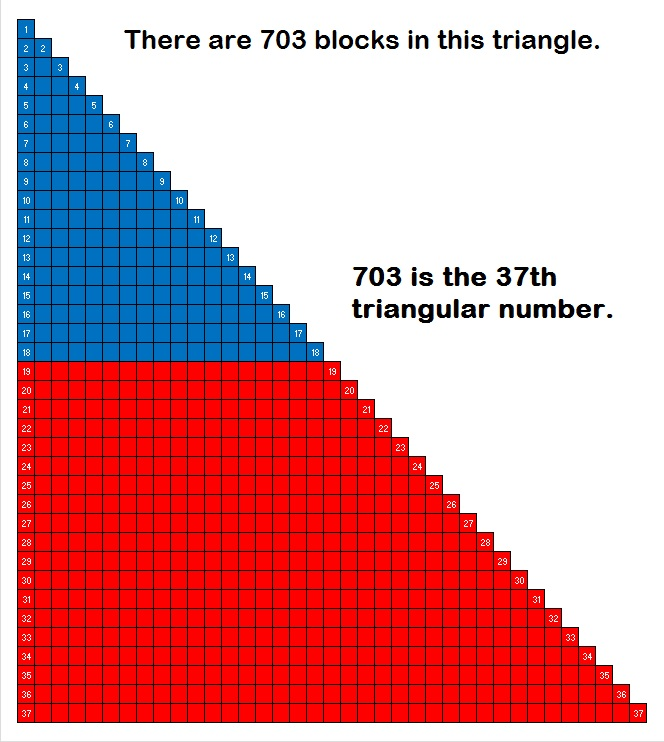703 is a triangular number because 37(38)/2 = 703. If we move part of the triangle, those blocks can be neatly rearranged into this rectangle:About half of all triangular numbers are also hexagonal numbers. 703 is the 19th hexagonal number because (19⋅2)(19⋅2 – 1)/2 = 703.

In the past I found counting the dots in graphics of larger hexagonal numbers to be a nightmare, but yesterday I saw a small graphic for hexagonal number 28 that used different colors for each expansion. I challenged myself to make a graphic showing that 703 is a hexagonal number. I made it in excel using o’s in different colors. When I finished, I told excel to replace the o’s with •’s. Excel informed me that it made 703 replacements.  Then I adjusted the size of the cells to make the hexagon smaller. I also attempted to make the outer-most hexagon look as symmetrical as possible.Hexagonal numbers are not symmetrical the way hexagonal snowflakes are. I thought it would be cool to make 703 into a snowflake, but I didn’t succeed because 703 is just too big and it lacks symmetry. Nevertheless, I must share these directions to make snowflakes that are indeed 6-sided. (Using paper dinner napkins instead of regular paper makes folding and cutting them much simpler.)

703 is a palindrome in three different bases:

• 383 base 14; note that 3(196) + 8(14) + 3(1) = 703
• 111 base 26; note that 1(676) + 1(26) + 1(1) =703
• JJ base 36; note that J is equivalent to 19 in base 10 and 19(36) + 19(1) = 703

Since 37 is one of its factors, 703 is the hypotenuse of the Pythagorean triple we see in this triangle:If you divide each number in the triple by 19, you’ll get the primitive Pythagorean triple 12-35-37.

703 is also a leg in some Pythagorean triple triangles:

• 504-703-865 (a primitive generated from 2(28)(9), 28² – 9², 28² + 9²) Note that 28 + 9 = 37 and 28 – 9 = 19. Both 37 and 19 are prime factors of 703.
• 703-6660-6697
• 703-12996-13015
• 703-247104-247105 (another primitive)# 630 Factor Trees and Level 2

### Today’s Puzzle: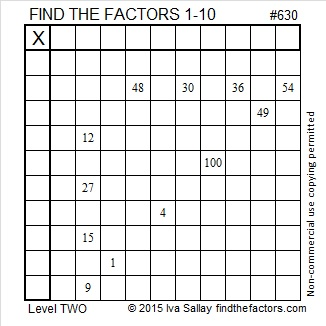Print the puzzles or type the solution on this excel file: 10 Factors 2015-09-28

### Factors for Today’s Puzzle: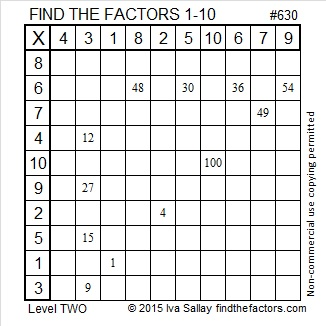### Factor Trees for 630:

630 is the 7th number with exactly 24 factors. So far, the seven numbers counting numbers with 24 factors are 360, 420, 480, 504, 540, 600, and 630. No counting number less than 630 has more than 24 factors.

Two of those seven numbers make up the Pythagorean triple 378-504-630. Which factor of 630 is the greatest common factor of those three numbers in the triple?

Here are a few of the MANY possible factor trees for 630.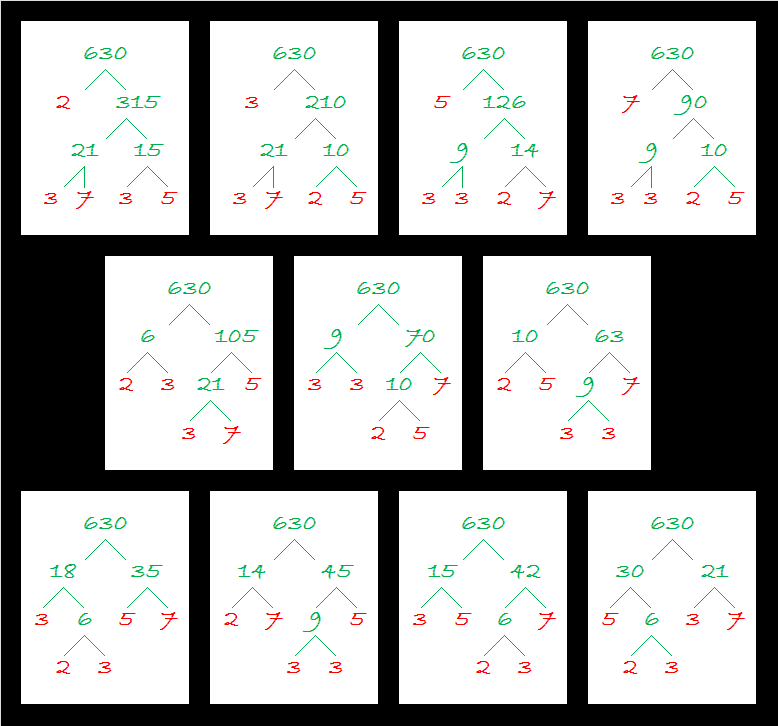### Factors of 630:

• 630 is a composite number.
• Prime factorization: 630 = 2 x 3 x 3 x 5 x 7, which can be written 630 = 2 x (3^2) x 5 x 7
• The exponents in the prime factorization are 1, 2, 1, and 1. Adding one to each and multiplying we get (1 + 1)(2 + 1)(1 + 1)(1 + 1) = 2 x 3 x 2 x 2 = 24. Therefore 630 has exactly 24 factors.
• Factors of 630: 1, 2, 3, 5, 6, 7, 9, 10, 14, 15, 18, 21, 30, 35, 42, 45, 63, 70, 90, 105, 126, 210, 315, 630
• Factor pairs: 630 = 1 x 630, 2 x 315, 3 x 210, 5 x 126, 6 x 105, 7 x 90, 9 x 70, 10 x 63, 14 x 45, 15 x 42, 18 x 35, or 21 x 30
• Taking the factor pair with the largest square number factor, we get √630 = (√9)(√70) = 3√70 ≈ 25.09980.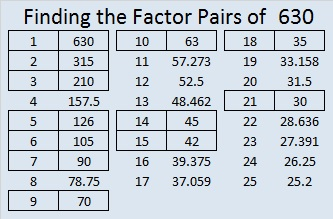### Sum-Difference Puzzle:

630 has twelve factor pairs. One of the factor pairs adds up to 53, and a different one subtracts to 53. If you can identify those factor pairs, then you can solve this puzzle!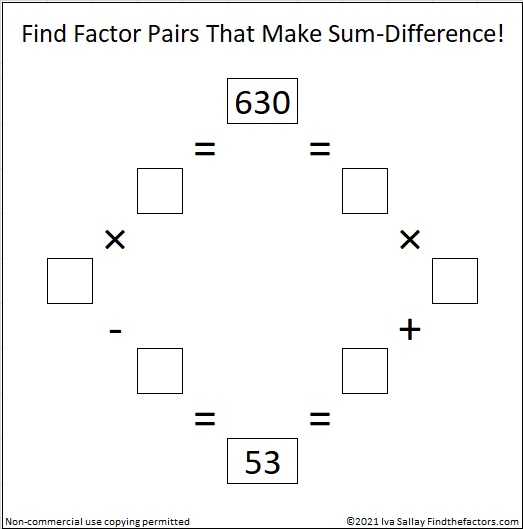### More about the Number 630:

630 is the sum of the six prime numbers from 97 to 113.

630 is the 35th triangular number because (35 x 36)/2 = 630. It is also the 18th hexagonal number because 18(2 x 18 – 1) = 630.

630 is a triangular number that is a multiple of other triangular numbers in more ways than you probably want to know:

• 630 is three times the 20th triangular number, 210, because 3(20 x 21)/2 = 630.
• 630 is 6 times the 14th triangular number, 105, because 6(14 x 15)/2 = 630.
• 630 is 14 times the 9th triangular number, 45, because 14(9 x 10)/2 = 630.
• 630 is 30 times the 6th triangular number, 21, because 30(6 x 7)/2 = 630.
• 630 is 42 times the 5th triangular number, 15, because 42(5 x 6)/2 = 630.
• 630 is 63 times the 4th triangular number, 10, because 63(4 x 5)/2 = 630.
• 630 is 105 times the 3rd triangular number, 6, because 105(3 x 4)/2 = 630.
• 630 is 210 times the 2nd triangular number, 3, because 210(2 x 3)/2 = 630.
• and finally, 630 is 630 times the 1st triangular number, 1, because 630(1 x 2)/2 = 630.